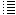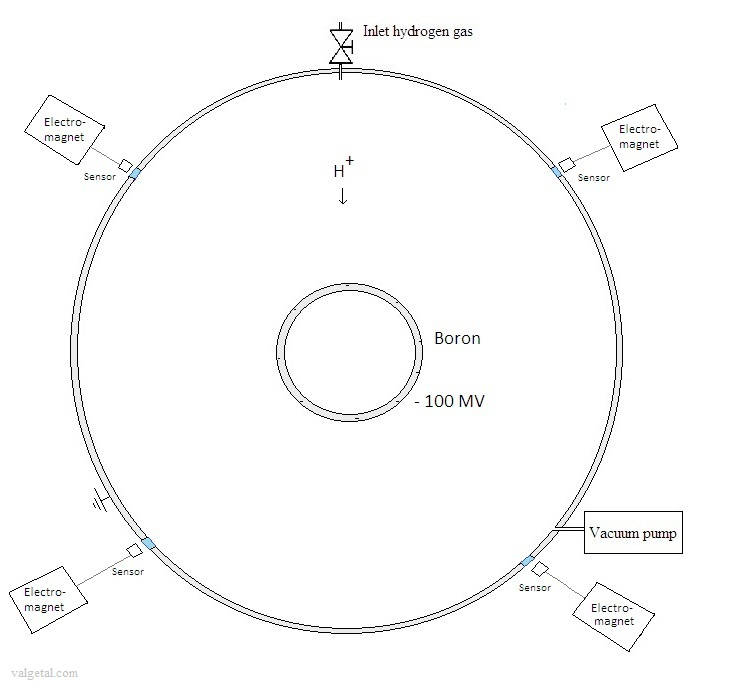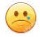7 Breaking the Coulomb barrier by electrostatic acceleration of ions in a device with a floating inner grid and a very high voltageJust some idea's... (will not produce net energy)

(7e idea)

Let's us consider the following:

Fig. 1. Schematic representation of an outer sphere and a floating inner sphere. (outer dimensions about 1 mtr diameter)The outer sphere is made of a conducting metal, strong enough to withhold a vacuum inside. The inside of the outer sphere is pumped vacuum.

The inner sphere is hollow and is made of a conducting and magnetic boron compound (or only the outside surface of it is made of boron and the inside consists of a conducting and magnetic metal). Some electromagnets are placed outside the outer sphere. The position of the inner sphere is monitored by sensors. With help of these sensors the electromagnets keep the inner sphere floating in the centre.

For an example of keeping an object floating by means of an electromagnet: my.execpc.com/~rhoadley/magsusp.htm

Suppose, by one way or another, the inner sphere has been charged negatively with a very high voltage: for example -100 MV.

Because there is a vacuum inside the outer sphere, the inner sphere is perfectly isolated: there cannot take place any discharge... or it can..?   Electrons can escape from a heated cathode for example. See also http://en.wikipedia.org/wiki/Field_electron_emission  Is it possible to accumulate so many electrons on the surface of the inner sphere to reach such a high voltage difference?

Let's suppose (hope) that this is possible.

---

Now we let in some hydrogen. Because of the very strong electric field it will be ionized. The positive hydrogen ions will be accelerated towards the negatively charged inner sphere.

With a voltage of - 100 MV, the ions will obtain an energy of 100 MeV.

Could it be possible perhaps that this would cause a fusion reaction when the hydrogen ion collide with the boron material of the inner sphere?

---

Let's consider the boron-hydrogen fusion reaction:

115B + 11H  -> 3 42He  + 8,68 MeV   (see en.wikipedia.org/wiki/Aneutronic_fusion)

No noxious neutrons are produced and the 3 positive  42He+ particles will move with high speed out of the centre increasing the voltage. (They could perhaps even be used to generate directly electricity.)

The strong nuclear force works until ± 2 fm = 2.10 -15 m  (see: wiki/Nuclear_force )

Z B  = 5  (amount of positive protons in a boron ion)

Z 1    = 1  (only one positive proton in a hydrogen ion)
k is the Coulomb's constant = 9,0×109 N m² C−2
1 eV = 1,6 . 10
-19  J
= >
Ucoul =  k . Z B . Z 1 . e 2  / r  =  9.0 . 10 9 . 5 . 1 . (1,6.10
-19 ) 2  / (2.10 -15)  = 0,6.10 -12  Nm =    0,6 .10 -12   J  =  3,6. MeV

(see: wiki/Coulomb_barrier )

So to break the coulomb barrier between a boron nucleus and hydrogen nucleus about 4  MeV energy is needed (note: according this very simple calculation).  If a hydrogen ion is accelerated from about the outer sphere to the surface of the inner sphere and achieves a kinetic energy of 100 MeV, this should be more than enough to cause a nuclear fusion reaction.  (If it would collide with a right angle with a boron nucleus...)

How to charge the inner sphere? Perhaps with an electron gun?

Fig. 2. Schematic representation of an outer sphere and a floating inner sphere with an electron gun.Electron guns are used in cathode ray tubes (televisions), for example . (Electron_gun)

We let the electrons travel inside a conducting tube (with holes). Inside this tube there will be no electric field, because it is a Faraday cage. Inside the inner sphere there will neither exist an electric field. This is a property of a hollow electric conducting sphere. Let's suppose that the hole under in the inner sphere is small and will not have significant influence.

When the electrons have arrived inside the inner sphere, they will not encounter any electric field, but yes mutual repulsion. In this way they will move to the outside surface of the inner sphere and increase the negative electric charge and voltage (similar as in Van der Graaff generator).

The nuclear fusion reactions will generate heat, which could be used to warm up water outside the outer sphere.

The inlet of hydrogen can be controlled, so the generating of heat as well.

---

Questions/doubts:

The magnetic fields will alter the path of the electrons... If the speed of the electrons is high enough and the magnetic fields are not so strong, than the electrons could maybe still manage to arrive in the inside of the inner sphere.

Can there exist a voltage difference so huge between the inner sphere and the conducting tube? (the distance between them is rather small). Perhaps it is better to isolate the conducting tube from the outer sphere.

Maybe not all collisions of hydrogen ions on the surface of the inner sphere will cause a fusion reaction. So what will be the efficiency?

The electric field inside the inner sphere will be really zero? To make the electric field zero inside the conducting tube there will accumulate positive charge on the final part of this tube near/inside the inner sphere. This could create an electric field inside the inner sphere...  Also, bringing electrons to a potential of - 100 mV  you can compare with bringing water to a very high altitude basin. In one way or another you have to overcome the height: the electrons have to overcome the potential difference. Otherwise we would create a kind of perpetuum mobile: the electrons travel without effort to the huge negative potential of -100 mV, and there they could release their energy by travelling back to the outer sphere. So just outside the conducting tube, where the electrons leave, there will be a huge electric field impeding the electrons to reach the inner sphere. This electric field can only be overcome by giving the electrons a very high speed (or what is the same, a very high energy). To give the electron this high speed you need in the electron gun a voltage difference of 100 mV, which is impossible..

Could there be another way to charge the inner sphere and give it a voltage of - 100 mV?

Maybe..,  see Eighth idea

Remark: If an ion passes a voltage difference of more then 100.10V it looses a lot of energy, more then the energy generated by a possible fusion... so this device will never generate a net energy..(Jan. 2013) (Jan. 2013) (Jan. 2013) (3 Febr. 2013) (6 Febr. 2013) (8 Febr. 2013) (16 Jan. 2014) (17 Jan. 2014) (20 Jan. 2014) ( Febr. 2014- 2016) Computer simulation tenth idea (2014-2016) Eleventh idea   (2016.. 2017)16 February 2014      by  Rinze Joustra        www.valgetal.com